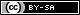# Dev Blog

 ./dev Original theme by orderedlist (CC-BY-SA) Where applicable, all content is licensed under a CC-BY-SA.# Shannon Entropy

Claude E. Shannon's book, "The Mathematical Theory of Communication", is very accessible. The main points about how entropy is defined and derived along with the "Fundamental Theorem for a Discrete Channel With Noise" is digested below.

## Entropy

Entropy can be defined as the number of bits it takes to describe a system.

Given $n$ symbols, each occurring with probability $p_k$ for $k \in (0, 1, \dots, n-1)$, we ask how many configurations are there for a very long message, say of $T$ transmitted symbols.

For the sake of clarity, we assume $T$ large and $T \cdot p_k \cdot n$ is integral.

The number of ways to arrange $T \cdot n$ elements comprised of $n$ symbols each occurring with $T \cdot p_k \cdot n$ frequency is the multinomial:

$${ T \cdot n \choose (T \cdot p_0 \cdot n), (T \cdot p_1 \cdot n), \dots, (T \cdot p_{n-1} \cdot n) }$$ $$= \frac{(T \cdot n)!}{\prod_{k=0}^{n-1} (T \cdot p_k \cdot n)!}$$

If we concern ourselves with the bits it takes to represent the total number of configurations, we find (where $\lg(\cdot) = \log_2(\cdot)$):

$$\lg( \frac{(T \cdot n)!}{\prod_{k=0}^{n-1} (T \cdot p_k \cdot n)!} )$$ $$= \lg( (T \cdot n)! ) - \sum_{k=0}^{n-1} \lg( (T \cdot p_k \cdot n)! )$$

$$\approx (T \cdot n) lg( T \cdot n ) - (T \cdot n) - \sum_{k=0}^{n-1} [ (T \cdot p_k \cdot n) \lg(T \cdot p_k \cdot n) - (T \cdot p_k \cdot n) ]$$

By definition, $\sum_{k=0}^{n-1} p_k = 1$, we can reduce to find:

$$= - T \sum_{k=0}^{n-1} p_k \lg(p_k)$$

We define $H$ to be our entropy, the average number of bits needed to represent our system. Since the above is the total number of bits needed, we divide by $T$ to find the average:

$$H = - \sum_{k=0}^{n-1} p_k \lg(p_k)$$

## Transmission Over a Noisy Channel

If we transmit $H$ bits per symbol over a noisy line and assume each symbol's error over the line is independent, label the number of bits, whole or partial, that succumb to error as $r$. That is, of the $H$ bits per symbol, $r$ are 'eaten' by noise in the channel.

Call the channel capacity $C = H - r$. This is the number of useful bits that remain after we take away the noise from the number of bits needed to encode symbols.

As above, consider a long message of $T$ transmitted symbols. First allocate some bits for error correction and choose $S$ such that:

$$S < C = H - r$$

Further

$$S = C - \eta = H - r - \eta$$

Where the number of error correcting bits is just shy of $T \cdot \eta$. Choose codewords in the source representation so that there are $2^{T \cdot S}$ codewords that sit in $2^{T \cdot H}$ total configurations.

Sent messages will be from the restricted set of codewords and has probability:

$$\frac{2^{T \cdot S}}{2^{T \cdot H}} = 2^{T \cdot (S - H)}$$

A received message of $T \cdot H$ bits long will have $T \cdot r$ corrupted by error. The number of possible source configurations that could have sent the received message is:

$$2^{T \cdot r}$$

The probability that there is another codeword in the $2^{T \cdot r}$ number of theoretical sent messages, aside from the source codeword, is the probability that none of the other codewords are hit:

$$[ 1 - 2^{ T \cdot (S - H) } ]^{ 2^{T \cdot r} }$$ $$= [ 1 - \frac{2^{ -T \cdot \eta}}{2^{T \cdot r}} ]^{2^{T \cdot r}}$$

As $T$ becomes large:

$$\approx e^{ -2^{ -T \cdot \eta } }$$ $$= 1 - 2^{ -T \cdot \eta } + O( 2^{-2 \cdot T \cdot \eta} )$$ $$\approx 1 - 2^{ -T \cdot \eta }$$

Which approaches 0.

So the chance of our transmitted encoded codeword being mistaken for another codeword is vanishingly small. As long as we choose $S$ to be less than the channel capacity $C$ and the message is long enough ($T$ is big enough) we have a low chance of a source codeword colliding after transmission with a channel error rate of $r$.